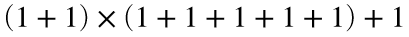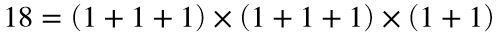# Problem 57487. Compute the largest number with a given integer complexity

Cody Problems 42831 and 42834 ask us to compute integer complexity, the smallest number of 1s needed to construct a number with addition and multiplication. For example, the integer complexity of 11 is 8 because it can be written as. The other numbers with integer complexity 8 are 13, 14, 15, 16, and 18. Thereforeis the largest integer with complexity 8.
Write a function to determine the largest number with given integer complexities. Return the answers as a cell array of strings.

### Solution Stats

32.0% Correct | 68.0% Incorrect
Last Solution submitted on Jul 18, 2023

### Community Treasure Hunt

Find the treasures in MATLAB Central and discover how the community can help you!

Start Hunting!